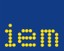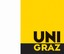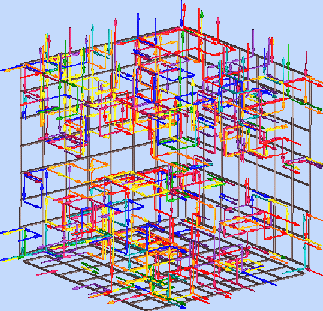##### Personal tools
You are here: Home Monopole Loops# QED Monopole Loops

Quantum Electro Dynamics (QED) is the quantum field theory of charged particles and photons. For mathematical definiteness it is formulated on a space-time grid, i.e. a 4D lattice. The quantization is performed by summing over many such quantum field configurations, weighted by a probabiltiy factor depending on the field configuration. As a first step only the photon field is quantized. Each such configuration consists out of a set of 4 complex (unit modulus) numbers for each space-time point of the lattice, the so-called link variables. (This is our "data" files.)

This form of QED has two phases with a phase transition between. In the hot phase (large electromagnetic coupling e) there is confinement of all charges (only chargeless bound states are allowed) and an abundance of monopole loops [This are to be found in our "mono" data sets]. In the cold phase (small coupling e) the photons are massless and there are few if any monopoles. This is the physical phase realized in nature.

Questions: Can one identify the phase transition, e.g. by „hearing“ the monopole density or other observables? Can one indeitfy the phase one is in for a specific coupling? Are such signals typical for sample configurations?(Picture Source: http://www.physik.uni-wuppertal.de/Studium/Lehrveranstaltungen/Computertheoretikum/Projekte/ct4/)

##### Document Actions